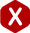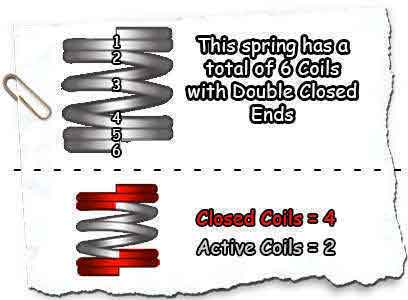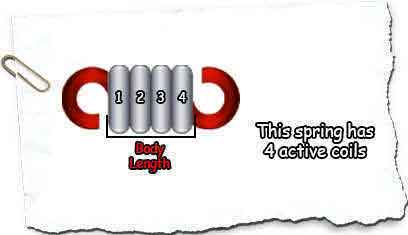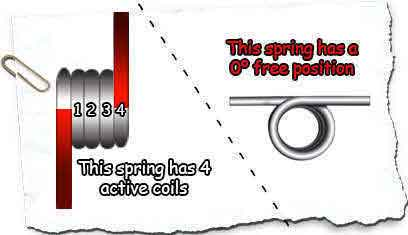<# Counting Active Coils

## Definition:

### Compression Spring Active Coils

Did you know that all the coils on a compression spring are not active? The energy a spring exerts comes from its active coils and how much pitch there is in between each coil. This means that if your coil compression spring has closed and squared ends, closed and ground ends, or double closed ends, it will have less active coils due to the fact that the coils on the ends are closed and have no pitch in between them thus giving you no force.

It is of great importance that you know the correct amount of total and active coils. Closed and squared ends along with closed and ground ends are closed coils. This means that if you have a total amount of 10 coils, you will have to subtract the two closed coils on the ends and calculate a total of 8 active coils. For double closed ends, there are two closed coils on each end so in this case instead of subtracting two closed coils from the 10 total coils, you will subtract four (4) closed coils to calculate a total of 6 active coils. In case that you have a spring with open ends, all coils are active due to the fact that they all have pitch in between.### Extension Spring Active Coils

Extension spring’s coils are all active. The way to determine the total amount of active coils your spring has is to divide the body length (length of spring without the hooks), divide it by the wire diameter, and subtract one coil. If you happen to have an extension spring with a 2.275” inch body length and a 0.025 wire diameter, divide 2.275 by 0.025 and subtract 1. This will give you a total of 90 active coils.### Torsion Spring Active Coils

Like extension springs, a torsion spring’s coils are all active. One thing you have to take into mind with torsion springs, though, is that there will usually be a fraction of a coil more or a fraction of a coil less. Why? Because the angle of the free position of your spring is very important so if your spring’s legs are not at a 0º angle, it means you have had to add a fraction of a coil more in order to meet your desired free position.